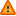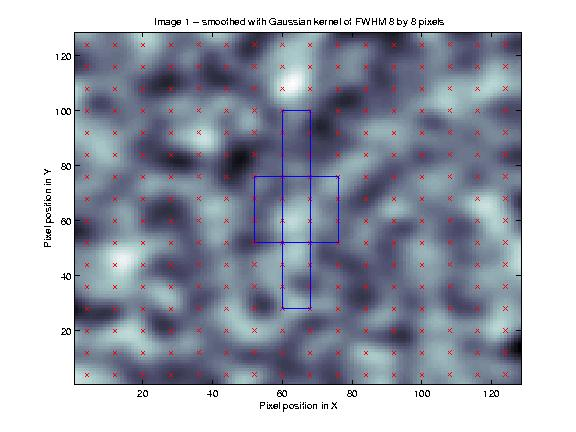SmallVolumeCorrection - MRC CBU Imaging WikiYou should trust both wikis because the password could be read by the particular administrators.

Clear message
location: SmallVolumeCorrection

# Small volume corrections using the theory of random fields

## Some notes to start

This file tries to explain the implemention of small volume random field corrections described in Worsley et al (1996). This paper is referred to as W96 in the rest of the document. For a basic introduction to random field theory, please see my random fields tutorial. The random fields tutorial explains various concepts used in this page, such as resels, and the Euler characteristic; if you are not familiar with these, I strongly suggest that you read that tutorial first. Matlab code to generate the figure is in http://imaging.mrc-cbu.cam.ac.uk/scripts/smallvoltalk.m.

The volume corrections here are implemented in SPM99 and later, mostly. I have also written some software for SPM99 and the (very old) SPM96 with some extra features - see the VolCorr page for details. For most purposes the implementation in SPM99 and later should be fine.

## Why use small volume corrections?

The formulae in W96 give p values corrected for multiple comparisons in Random Field (RF) images, which are applicable for volumes of any size or shape. SPM96 used earlier results from RF theory to give an approximate p value for the chance of observing a given maximum statistic (Z or F) of given magnitude, if the maximum had been taken from a large given volume of similar (Z or F) statistics, with a given smoothness (FWHM) in three dimensions. This p value is therefore the chance of observing such a maximum on the null hypothesis that the entire statistic map is without underlying effect, and therefore random. The corrected p value corresponds to the P(Zmax>u) column in the standard SPM96 printout - the correction for "height". For volumes which are large compared to the smoothness (FWHM), this corrected p value is very close to that according to the newer results of W96.

SPM99 and later versions use the W96 results to calculate corrected statistics across the whole brain, by working out the shape and size of the whole brain volume in the analysis, and calculating the correction accordingly.

Thus, for whole-brain analyses, even SPM96 offered a reasonable correction for the entire brain volume. For smaller volumes, W96 shows that the correction must take into account the geometric properties of the volume, such as shape and surface area.

This is important, because you may well have an apriori hypothesis as to an area of expected activation in a statistical parametric map. In such a case, to correct for multiple comparisons across the whole image is too conservative, as you are restricting your interest to a subset of the comparisons being made. The W96 paper gives results which allow the choice of appropriate thresholds given that you are restricting your investigation to a certain volume of interest (VOI), of defined shape, size, etc. This is because both size, and shape, dictate how many resels the volume will contain. As the random fields tutorial explains, the number of resels in a volume is a measure related to the number of independent observations in that volume, and this in turn will dictate how strict our correction must be.

## Why does size matter?

This is best illustrated using a figure. The figure below is based on one of the figures from my random fields tutorial. I have taken a set of 128 by 128 independent random numbers, and smoothed them with a kernel of 8 pixels FWHM (see my smoothing tutorial for an explanation of this procedure).The centre of each resel is marked with a cross. In the centre of the figure is a square with sides that are 24 pixels long. This square, including its edges, contains 4 x 4 = 16 crosses (resel centres). Given that resels are related to independent observations, a larger square will contain more resels, and therefore have more independent observations.

## Why shape?

Different shapes will contain data from different numbers of resels. In the figure above, there is also a long thin box, which is of the same volume as the square. This shape abuts on 18 resel centres, so that, even for the same volume, the resel correction will depend on the shape.

## How do I use this correction?

Some of the formulae from W96 are implemented in SPM99 and later. They are used to calculate the whole brain random field corrections, and for the output of the SVC (Small Volume Correction) button, in the input window after you have entered the results section. This button will give you p values (expected Euler characteristics) for voxels contained within boxes or spheres centred around the current selected voxel in the results window, or for voxels within a masking image (see the Creating VVOI images section for more details). An image is useful when you have an apriori hypothesis about where the activation is, that is not a box shape or a sphere. We may therefore need to define our search region to be a more complex shape; this shape might correspond to an anatomical region, or the results of an activation from a previous experiment.

## Choosing appropriate volumes of interest

The p values given by using the box, sphere or VVOIs are approximate. In particular, for the VVOIs, the approximation is most accurate when the shape is convex, such as an ellipsoid. Complex non-convex shapes, such as an unsmoothed gray matter map, may have a large surface area compared to its volume, leading to higher thresholds than may be appropriate (see W96 for discussion). In addition, VVOIs with curved edges will appear to have a larger surface area than corresponding continuous shapes. This is because the surface area is inflated by the lego-brick effect at the VVOI edges. For this reason, on the suggestion of Keith Worsley, SPM applies a correction to the calculated surface area and diameter of VVOIs, on the basis that most regions specified with VVOIs are likely to have curved edges. When the VVOI has little or no lego-brick effect - for example when the VVOI is a perfect box shape - the surface area correction for VVOIs will not be appropriate, and the "box" specification option is better. Note that the calculations always assume 3D VOIs. Calculations for 2D shapes should be simple to implement given the underlying code.

## Creating VVOI images

Images for VVOI calculation need to be the same shape, size and orientation as the region of interest in the statistic image. They must have the same orientation in X Y and Z, in voxel space (.mat files and origin values are ignored). Any voxels in the VVOI image that contain values not exactly equal to zero are taken to be within the VVOI.There are two common reasons to create such VVOI images. The first is that you wish to base your VVOI on a thresholded statistical image that is orthogonal to the statistical map for which you wish to derive corrected p values. You might for example have done an earlier experiment that gave an statistical map showing areas of activation, and want to look only within these areas in your current analysis. In such a case you could use SPM to generate the image, by doing the following: use the results section of SPM to display the first statistical map thresholded as required. For SPM99 and later click on the 'write filtered' button in the input window. SPM asks you for an output filename for the thresholded image. This image will contain the test statistic value in the areas surviving the thresholding, and zeros elsewhere, and you can use this image to define the VVOI.

The second common situation is when you wish to look at some predefined anatomical area or set of areas. To create an image defining these areas you will need an ROI drawing package that can write binary images, such as Chris Rorden's freeware MriCro package for the PC.

You can check that the VVOI covers the regions that you expect using the SpmCheckReg button in SPM99.

## Some last technical notes

Note that, for the purposes of the VVOI calculations, the voxel values are taken to represent points in a lattice, rather than touching cubes (see W96). Thus a VVOI cube of 6 x 6 x 6 voxels, with 2 x 2 x 2 mm voxel size, will be calculated to have a 10 x 10 x 10 mm volume. The VVOI image does not have to have the same voxel dimensions or voxel size as the statistic image, only it must be the same shape, size and orientation as the region of interest in the statistic image. Non-zero voxels at the edge of the VVOI image are taken to be at the edge of the VVOI. For example, let us imagine that I wanted to define a cube-shaped VVOI of 12 x 12 x 12 mm (in practice this would not be a good idea, because of the surface area correction applied to VVOIs - see above). My statistic image might be 60 x 70 x 60 voxels (which is irrelevant), with voxel size 2 x 2 x 2 mm. A correct VVOI could be (rather bizarrely) defined by saving an image containing only 13 x 13 x 13 voxels, all non-zero, with 1 x 1 x 1 mm voxel size.

MatthewBrett 29/9/99

## Reference

Worsley, K.J., Marrett, S., Neelin, P., Vandal, A.C., Friston, K.J., and Evans, A.C. (1996). A unified statistical approach for determining significant signals in images of cerebral activation. Human Brain Mapping, 4:58-73.